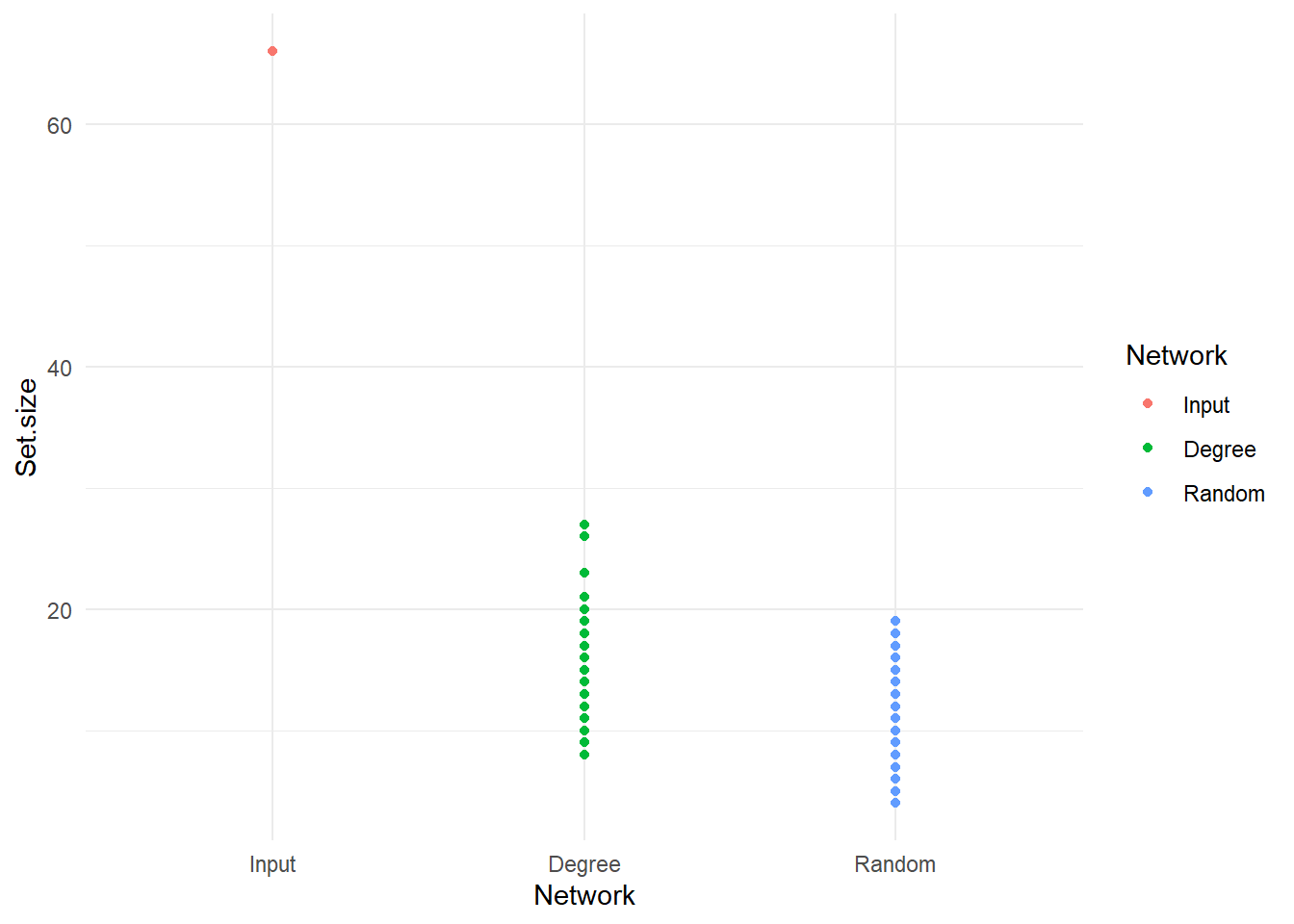# Network analysis - conserved patterns

In this tutorial, we will look at the Axinellida network with anuran.
You can install anuran following the instructions on the Github: anuran Github repository.

## Step 1 - Data

For this tutorial, we will use the networks from the FlashWeave tutorial: Running FlashWeave on sponge networks.
If you were unable to run FlashWeave, please find the networks here: ZIP file with networks.

## Step 2 - Running anuran on the sponge networks

Although all networks look superficially similar, it would be nice to have some idea of just how similar they are. For example, are the most central nodes in these networks identical? We can check such network properties with anuran.

Like before, first check your installation.

anuran -h

This software accepts (multiple) groups of networks. It will try to import all networks in a folder and run analyses on these. Therefore, make sure the sponge_networks folder only contains the graphml or gml networks and give anuran the name of the folder. We can get some initial figures with the -draw flag. Do you think that the networks are very similar? How big is the overlap? Solution.

anuran -i folder -o demo -draw

What parameter should you add to change the intersections? Rerun anuran with partial intersections before moving to the next section. Solution.

With the default plotting, the y-axis is a bit inconvenient because the difference is so much larger than the intersection. We can import the csv file and plot the intersection with ggplot2.

library(ggplot2)

data <- data[data$Set.type == 'Intersection 0.3',] data$Network <- factor(data\$Network, c('Input', 'Degree', 'Random'))
ggplot(data, aes(x=Network, y=Set.size, colour=Network)) + geom_point() + theme_minimal()Can you change the figure above so it shows how the number of shared edges changes from 2 to 10 networks? Solution.

The intersection sizes that are generated, are a function of the matching edges across networks. Networks can be similar even if the edges are not. For example, nodes that occupy a central position in one network may also be central in the second network, even if they share no neighbours. We only need to add a single flag to study node centralities with anuran. We will also reduce the number of null models and iterations, so it runs a bit faster.

anuran -i folder -o demo -draw -c -size 0.2 0.3 0.5 0.7 1 -perm 5 -nperm 10 

With R, we can import the csv file that was just generated.

centralities <- read.csv('demo_centralities.csv', stringsAsFactors = FALSE, row.names=1)
knitr::kable(head(centralities[,1:9], 3))
Node Network Group Network.type Conserved.fraction Prevalence.of.conserved.fraction Centrality Upper.limit Lower.limit
0 c92920 Input sponges_networks Input networks NA NA Degree 0.7596989 0.2887912
1 c253327 Input sponges_networks Input networks NA NA Degree 0.5041260 0.0000000
2 c4361909 Input sponges_networks Input networks NA NA Degree 0.6774391 0.2168139

The table above shows the first three values of the csv file. In this case, it shows for three taxa the 95% confidence interval of the degree (Upper.limit and Lower.limit). The centralities per network are also included in the table (but not shown), and can be useful after some parsing.

In addition to the option to evaluate conserved edges or centralities across networks, anuran includes an option to add positive controls. Can you run anuran with positive controls and try visualizing these as well? Solution.

Back to overview